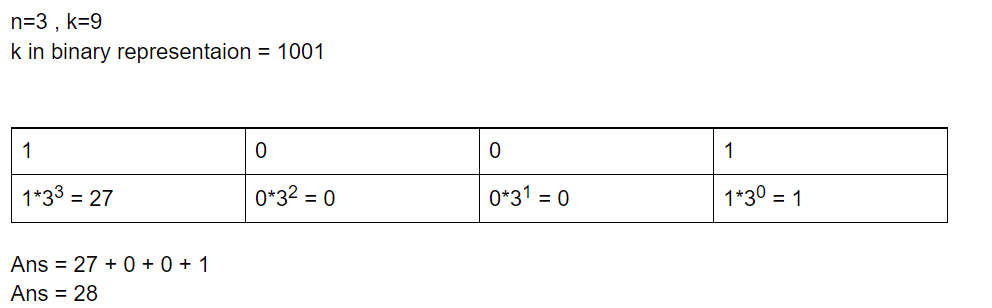# Find Kth number that can be written as sum of different powers of N

• Difficulty Level : Medium
• Last Updated : 26 Oct, 2021

Given two positive integers N and K. The task is to find the Kth number that can be written as the sum of different non-negative powers of N

Examples:

Attention reader! Don’t stop learning now. Get hold of all the important mathematical concepts for competitive programming with the Essential Maths for CP Course at a student-friendly price. To complete your preparation from learning a language to DS Algo and many more,  please refer Complete Interview Preparation Course.

Input: N = 3, K = 4
Output: 9
Explanation:
First number that can be writeen as sum of powers of 3 is [1 = 30]
Second number that can be writeen as sum of powers of 3 is [3 = 31]
Third number that can be writeen as sum of powers of 3 is [4 = 30 + 31]
Fourth number that can be writeen as sum of powers of 3 is [9 = 32]

Input: N = 2, K = 12
Output: 12

Approach: This problem can be solved by using the concept of decimal to binary conversion.  The idea is to find the binary representation of K and start iterating from the least significant bit to the most significant bit. If the current bit is set then include the corresponding power of N to the answer otherwise skip that bit. Refer to the picture below for a better understanding.Example: When N = 3, K = 9

Below is the implementation of the above approach:

## C++

 `// C++ program for the above approach``#include ``using` `namespace` `std;` `// Function to find Kth number that can be``// represented as sum of different``// non-negative powers of N``int` `FindKthNum(``int` `n, ``int` `k)``{``    ``// To store the ans``    ``int` `ans = 0;` `    ``// Value of current power of N``    ``int` `currPowOfN = 1;` `    ``// Iterating through bits of K``    ``// from LSB to MSB``    ``while` `(k) {``        ``// If the current bit is 1 then include``        ``// corresponding power of n into ans``        ``if` `(k & 1) {``            ``ans = ans + currPowOfN;``        ``}` `        ``currPowOfN = currPowOfN * n;``        ``k = k / 2;``    ``}` `    ``// Return the result``    ``return` `ans;``}` `// Driver Code``int` `main()``{``    ``int` `N = 3;``    ``int` `K = 4;` `    ``cout << FindKthNum(N, K);``}`

## Java

 `// Java program for the above approach``class` `GFG``{``  ` `  ``// Function to find Kth number that can be``  ``// represented as sum of different``  ``// non-negative powers of N``  ``static` `int` `FindKthNum(``int` `n, ``int` `k)``  ``{``    ` `      ``// To store the ans``      ``int` `ans = ``0``;` `      ``// Value of current power of N``      ``int` `currPowOfN = ``1``;` `      ``// Iterating through bits of K``      ``// from LSB to MSB``      ``while` `(k > ``0``)``      ``{``        ` `          ``// If the current bit is 1 then include``          ``// corresponding power of n into ans``          ``if` `((k & ``1``) == ``1``) {``              ``ans = ans + currPowOfN;``          ``}` `          ``currPowOfN = currPowOfN * n;``          ``k = k / ``2``;``      ``}` `      ``// Return the result``      ``return` `ans;``  ``}`` ` `  ``// Driver Code``  ``public` `static` `void` `main(String []args)``  ``{``      ``int` `N = ``3``;``      ``int` `K = ``4``;` `      ``System.out.println(FindKthNum(N, K));``  ``}` `}` `// This Code is contributed by ihritik`

## Python3

 `# Python program for the above approach` `# Function to find Kth number that can be``# represented as sum of different``# non-negative powers of N``def` `FindKthNum(n, k):` `  ``# To store the ans``  ``ans ``=` `0` `  ``# value of current power of N``  ``currPowOfN ``=` `1` `  ``# Iterating through bits of K``  ``# from LSB to MSB``  ``while` `(k > ``0``):``    ` `    ``# If the current bit is 1 then include``    ``# corresponding power of n into ans``    ``if` `((k & ``1``) ``=``=` `1``) :``      ``ans ``=` `ans ``+` `currPowOfN``    `  `    ``currPowOfN ``=` `currPowOfN ``*` `n``    ``k ``=` `k ``/``/` `2``  `  `  ``# Return the result``  ``return` `ans`   `# Driver Code` `N ``=` `3``K ``=` `4``print``(FindKthNum(N, K));``  `  `# This Code is contributed by ihritik`

## C#

 `// C# program for the above approach``using` `System;``class` `GFG``{``  ` `  ``// Function to find Kth number that can be``  ``// represented as sum of different``  ``// non-negative powers of N``  ``static` `int` `FindKthNum(``int` `n, ``int` `k)``  ``{``    ` `      ``// To store the ans``      ``int` `ans = 0;` `      ``// Value of current power of N``      ``int` `currPowOfN = 1;` `      ``// Iterating through bits of K``      ``// from LSB to MSB``      ``while` `(k > 0)``      ``{``        ` `          ``// If the current bit is 1 then include``          ``// corresponding power of n into ans``          ``if` `((k & 1) == 1) {``              ``ans = ans + currPowOfN;``          ``}` `          ``currPowOfN = currPowOfN * n;``          ``k = k / 2;``      ``}` `      ``// Return the result``      ``return` `ans;``  ``}`` `  `  ``// Driver Code``  ``public` `static` `void` `Main()``  ``{``      ``int` `N = 3;``      ``int` `K = 4;` `      ``Console.WriteLine(FindKthNum(N, K));``  ``}` `}` `// This Code is contributed by ihritik`

## Javascript

 ``

Output:
`9`

Time Complexity: O(log2K)

Space Complexity: O(1)

My Personal Notes arrow_drop_up